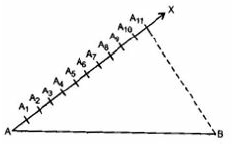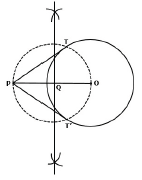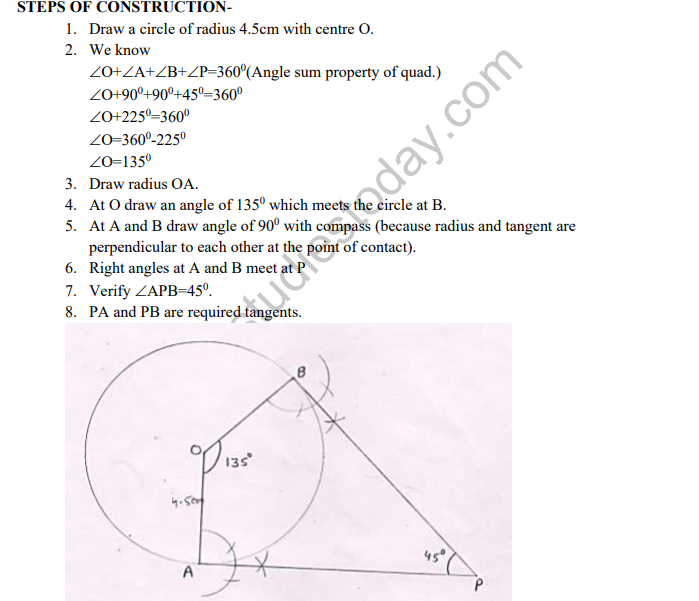# CBSE Class 10 Mathematics Constructions Assignment Set A

Read and download free pdf of CBSE Class 10 Mathematics Constructions Assignment Set A. Get printable school Assignments for Class 10 Mathematics. Standard 10 students should practise questions and answers given here for Chapter 12 Areas Related To Circles Mathematics in Grade 10 which will help them to strengthen their understanding of all important topics. Students should also download free pdf of Printable Worksheets for Class 10 Mathematics prepared as per the latest books and syllabus issued by NCERT, CBSE, KVS and do problems daily to score better marks in tests and examinations

## Assignment for Class 10 Mathematics Chapter 12 Areas Related To Circles

Class 10 Mathematics students should refer to the following printable assignment in Pdf for Chapter 12 Areas Related To Circles in standard 10. This test paper with questions and answers for Grade 10 Mathematics will be very useful for exams and help you to score good marks

### Chapter 12 Areas Related To Circles Class 10 Mathematics Assignment

Construction

Q.- To divide a line segment PQ in the ration 7 : 3 internally, first a ray PX is drawn so that ∠QPX is an acute angle and then at equal distances, points are marked on the ray PX such that the minimum number of these points is :
a. 7
b. 3
c. 4
d. 10

Ans-  d. 10
Explanation: According to the question, the minimum number of those points which are to be marked should be (Numerator + Denominator) i.e., 7 + 3 = 10

Q.- Which theorem criterion we are using in giving the justification of the division of a line segment by usual method?
a. Basic Proportionality theorem
b. SSS criterion
c. Pythagoras theorem
d. Area theorem

Ans- a. Basic Proportionality theorem
Explanation: The intercept theorem, also known as Thales' theorem or basic proportionality theorem, is an important theorem in elementary geometry about the ratios of various line segments that are created if two intersecting
lines are intercepted by a pair of parallels. It is equivalent to the theorem about ratios in similar triangles.

Q.-  To divide a line segment AB internally in the ratio 4 : 7, first a ray AX is drawn so that ∠BAXis an acute angle and then at equal distances, points are marked on ray AX such that the minimum number of these points are:
a. 9
b. 11
c. 10
d. 12

Ans- b. 11
Explanation: According to the question, the minimum number of those points which are to be marked should be (Numerator + Denominator) i.e. 4 + 7 = 11Q.- The construction of a triangle, similar and larger to a given triangle as per given scale factor m:n is possible only when
a.m<n
b.m=n
c. Independent of scale factor
d.m>n

Ans- d. m > n
Explanation: The construction of a triangle, similar and larger to a given triangle as per given scale factor, m:n is possible only when. Because We have to construct a similar and larger triangle so that side of this triangle should be larger to the given triangle.

Q.- To divide a line segment AB in the ration 4 : 7, a ray AX is drawn first such that ∠ BAX is an acute angle and then points …. are located at equal distances on the ray AX and the point B is joined to
a. A12
b. A10
c. A9
d. A11

Ans-  d. A11
Explanation: To divide a line segment AB in the ratio m:n (when m > n), a ray AX is drawn such that ∠ BAX is an acute angle and then points A1, A2, A3...Am, …..An…. are located at equal distances on the ray AX and then the point B is joined to

Q.- To draw a pair of tangents to a circle which are at right angles to each other, it is required to draw tangents at end points of the two radii of the circle, which are inclined at an angle of
a. 45o
b. 120o
c. 60o
d. 90o

Ans- d. 90oExplanation: According to the question, the tangents are inclined at an angle of 180o - 90o = 90o

Q.- Draw two tangents to a circle of radius 3.5 cm from a point P at a distance of 6.2 cm from its centre.

Ans-Steps of construction:
1. Take a point O on the plane of the paper and draw a circle of radius 3.5 cm.
2. Mark a point P at a distance of 6.2 cm from the centre O and join OP.
3. Draw a right bisector of OP, intersecting OP at Q.
4. Taking Q as centre and OQ = PQ as radius, draw a circle to intersect the given circle at T and T'.
5. Join PT and PT' to get the required tangents.

Level 3

Q1- Draw a pair of tangents to a circle of radius 4.5cm which are inclined to each other at an angle of 450Q2- Draw a circle of radius 6cm, draw a tangent to this circle making an angle of 300 with a line passing through the centre.